## 1. Introduction

In this article, we’ll describe how inheritance works in Scala and its various flavors. To clarify, inheritance is the mechanism that allows us to extend classes. In other words, it’s the way to access or override members and features of a superclass from a subclass.

## 2. Single Inheritance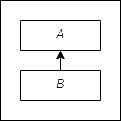Single inheritance is the most simple form of inheritance. As shown in the example below, one subclass extends only one superclass directly.

Let’s see the code:

``````class A() {
def name: String = "A"
}

class B() extends A() {
override def name: String = "B"
}``````

## 3. Multiple Level Inheritance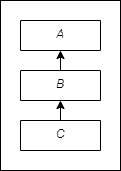Multiple level inheritance is the type of inheritance in which a subclass inherits from more than one superclass indirectly. As a result, the subclass can access members from its own superclass, from the superclass of its superclass, and so on:

``````object Multilevel {
class A() {
val a = "A"
}

class B() extends A() {
val b = "B"
}

class C() extends B() {
val c = "C"
}

def main(args: Array[String]): Unit = {
val c = new C
println(s"c.a = \${c.a}") // A property (non direct superclass)
println(s"c.b = \${c.b}") // B property (direct superclass)
println(s"c.c = \${c.c}") // C property
}
}``````

## 4. Multiple Inheritance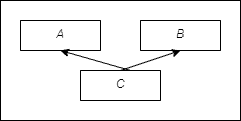Multiple inheritance is the type of inheritance where the subclass inherits directly from more than one class. In Scala, this is not achievable with classes. Instead, multiple inheritance is supported via traits.

Let’s write an example:

``````object Multiple {
trait A {
val a = "A"
}

trait B {
val b = "A"
}

class C extends A with B {
val c = "C"
}

def main(args: Array[String]): Unit = {
val c = new C
}
}``````

## 5. Hierarchical Inheritance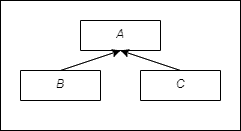In hierarchical inheritance, more than one class derives from the same superclass.

Let’s put it in code:

``````object Hierarchical {
class A() {
val a = "A"
}

class B() extends A() {
val b = "B"
}

class C() extends A() {
val c = "C"
}

def main(args: Array[String]): Unit = {
val b = new B
val c = new C
println(s"b.a = \${b.a}")
println(s"b.a = \${b.b}")
println(s"c.a = \${c.a}")
println(s"c.c = \${c.c}")
}
}``````

## 6. Hybrid Inheritance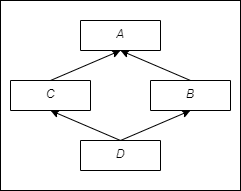Hybrid inheritance is any combination of two or more of the above inheritance types. In the following example, we demonstrate the classic diamond problem:

``````object Hybrid {
trait A {
val a = "A"
}

trait B extends A {
val b = "B"
override val a: String = "b.a"
}

trait C extends A {
val c = "C"
override val a: String = "c.a"
}

trait D extends B with C
trait E extends C with B

def main(args: Array[String]): Unit = {
val d = new D {}
val e = new E {}
println(d.a) // prints c.a
println(e.a) // prints b.a
}
}``````

In Scala, we can’t define classes that derive from more than one class: This is achievable only with traits.

It’s important to note that the trait methods are resolved from left to right. In other words, if two or more traits define the same method, then the method will be overridden by the last trait in the class definition.

## 7. Conclusion

In this tutorial, we presented Scala inheritance types with examples. Despite the fact that we didn’t use abstract classes in our examples, some of the above classes or traits could be defined as abstract classes. We’ve previously written about when to use abstract classes vs traits.

As always, the code of the above examples is available over on GitHub.